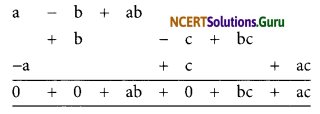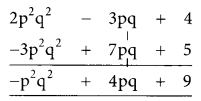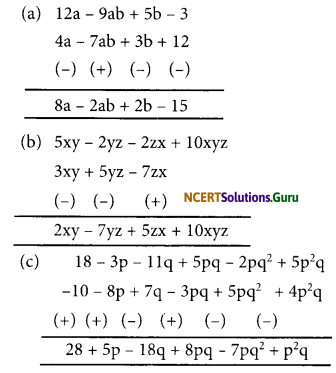# NCERT Solutions for Class 8 Maths Chapter 9 Algebraic Expressions and Identities Ex 9.1

These NCERT Solutions for Class 8 Maths Chapter 9 Algebraic Expressions and Identities Ex 9.1 Questions and Answers are prepared by our highly skilled subject experts.

## NCERT Solutions for Class 8 Maths Chapter 9 Algebraic Expressions and Identities Exercise 9.1

Question 1.
Identify the terms, their coefficients for each of the following expressions.
(i) 5xyz2 – 3zy
(ii) 1 + x + x2
(iii) 4x2y2 – 4x2y2z2 + z2
(iv) 3 – pq + qr – rp
(v) $$\frac{x}{2}+\frac{y}{2}$$ – xy
(vi) 0.3a – 0.6ab + 0.5b
Solution:
(i) 5xyz2 – 3zy
Terms are 5xyz2 and -3zy
Coefficients of 5xyz2 is 5
Coefficients of-3zy is -3

(ii) 1 + x + x2
Terms are x2, x, and 1
Coefficient of x2 is 1
coefficient of x is 1
and constant term is 1(iii) 4x2y2 – 4x2y2z2 + z2
Terms are 4x2y2, -4x2y2z2 and z2
Coefficient of x2y2 is 4
Coefficient of -4x2y2z2 is -4
Coefficient of z2 is 1

(iv) 3 – pq + qr – rp
Terms are 3, -pq, qr and -rp
Coefficient of 3 is 3.
Coefficient of -pq is -1.
Coefficient of qr is 1 and coefficient of -rp is -1

(v) $$\frac{x}{2}+\frac{y}{2}$$ – xy
Terms are $$\frac{x}{2}, \frac{y}{2}$$ and -xy
Coefficient of $$\frac{\mathrm{x}}{2}$$ is $$\frac{1}{2}$$
Coefficient of $$\frac{\mathrm{x}}{2}$$ is $$\frac{1}{2}$$
and coefficient of -xy is -1

(vi) 0.3a – 0.6ab + 0.5b
Terms are 0.3a, -0.6ab and 0.5b
Coefficient of 0.3a is 0.3
Coefficient of -0.6ab is -0.6
Coefficient of 0.5b is 0.5Question 2.
Classify the following polynomial as monomials, binomials, trinomials. Which polynomials do not fit in any of these three categories?
x + y, 1000, x + x2 + x3 + x4, 7 + y + 5x, 2y – 3y2, 2y – 3y2 + 4y3, 5x – 4y + 3xy, 4z – 15z2, ab + bc + cd + da, pqr, p2q + pq2, 2p + 2q
Solution:
Monomials: 100pqr
Binomials: x + y; 2y – 3y2; 4z – 15z2; p2q + pq2; 2p + 2q
Trinomials: 7 + y + 5x; 2y – 3y2 + 4y3; 5x – 4y + 3xy
Polynomials that do not fit in these categories: x + x2 + x3 + x4 and ab + bc + cd + da
(Since the above polynomials has four terms)

Question 3.
(i) ab – bc, bc – ca, ca – ab
(ii) a – b + ab, b – c + bc, c – a + ac
(iii) 2p2q2 – 3pq + 4, 5 + 7pq – 3p2q2
(iv) l2 + m2, m2 + n2, n2 + l2, 2lm + 2mn + 2nl
Solution:
(i) ab – bc; bc – ca; ca – ab(ii) a – b + ab; b – c + bc; c – a + ac(iii) 2p2q2 – 3pq + 4; 5 + 7pq – 3p2q2(iv) l2 + m2; m2 + n2; n2 + l2; 2lm + 2mn + 2nl= 2(l2 + m2 + n2 + lm + mn + nl)

Question 4.
(a) Subtract 4a – 7ab + 3b + 12 from 12a – 9ab + 5b – 3
(b) Subtract 3xy + 5yz – 7zx from 5xy – 2yz – 2zx + 10xyz
(c) Subtract 4p2q – 3pq + 5pq2 – 8p + 7q – 10 from 18 – 3p – 11q + 5pq – 2pq2 + 5p2q
Solution:error: Content is protected !!